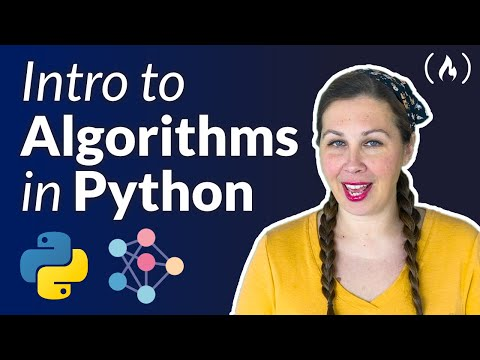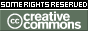# [#Script #Coding] Algorithms in Python – Full Course for Beginners

### Algorithms in Python – Full Course for Beginners

By freeCodeCamp.org
Published: Sep 22, 2022In this Introduction to Algorithms in Python course, you’ll learn about algorithm basics like recursion and then go all the way to working with concepts like dynamic programming.

The 5 main course segments include simple recursive algorithms, data structures, divide & conquer, greedy algorithms, and dynamic programming.

Joy Brock developed this course in collaboration with realtoughcandy.io. Check out Joy’s channel: https://www.youtube.com/c/PythonLearn…

Course Contents

• (0:00:00) Intro & course overview
• (0:07:07) Factorials refresher
• (0:10:04) CODING CHALLENGE: Factorial program using iteration, recursion
• (0:14:57) What is a permutation?
• (0:16:34) CODING CHALLENGE: Recursive permutation
• (0:20:13) Iterative permutation example
• (0:22:17) 8/N queens problem: theory & explanation
• (0:23:57) Real world example of permutations
• (0:24:49) Lesson recap
• (0:26:11) What are data structures?
• (0:27:53) What is a one-dimensional array?
• (0:29:01) Search & sort
• (0:30:39) CODING CHALLENGE: Linear search
• (0:31:00) Binary search
• (0:32:06) CODING CHALLENGE: Iterative binary search
• (0:33:31) Coding a recursive binary search
• (0:34:47) Bubble sort
• (0:36:42) CODING CHALLENGE: Bubble sort
• (0:38:02) Insertion sort
• (0:39:24) CODING CHALLENGE: Insertion sort
• (0:52:12) Hash tables
• (0:56:27) Lesson recap
• (0:57:42) Divide & conquer algorithm paradigm: uses, benefits and more
• (1:00:43) Merge sort
• (1:02:48) CODING CHALLENGE: An efficient merge sort
• (1:05:48) Getting judged mercilessly on LeetCode
• (1:06:47) Getting Python to do the work for us with sorted()
• (1:07:33) Matrix multiplication
• (1:10:06) CODING CHALLENGE: Matrix multiplication
• (1:11:20) Strassen algorithm
• (1:14:45) CODING CHALLENGE: Strassen algorithm
• (1:16:27) Lesson recap
• (1:17:21) What is a greedy algorithm?
• (1:19:20) Assign mice to holes conceptual overview
• (1:21:45) CODING CHALLENGE: Assign mice to holes
• (1:23:10) Fractional knapsack
• (1:23:36) Understanding the fractional knapsack problem with a (light-hearted) dystopian apocalypse example
• (1:25:54) Coding challenge prep
• (1:27:13) CODING CHALLENGE: Fractional knapsack
• (1:31:49) Egyptians fractions
• (1:34:03) CODING CHALLENGE: Egyptian fractions
• (1:37:06) Lesson recap
• (1:38:15) What is dynamic programming (also called DP)?
• (1:41:55) What is the principle of optimality?
• (1:42:20) The 3-step process to solving a problem with optimal substructure
• (1:43:55) Introduction to “ugly numbers”
• (1:47:19) CODING CHALLENGE: Ugly numbers
• (1:51:41) Traveling salesman problem (TSP)
• (1:55:49) CODING CHALLENGE: Traveling salesman problem
• (1:59:52) Palindromic matrix paths
• (2:03:11) CODING CHALLENGE: Palindromic matrix paths
• (2:08:37) Lesson recap
• (2:10:03) Course wrap up (and the importance of coding every day)